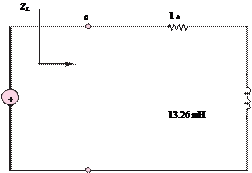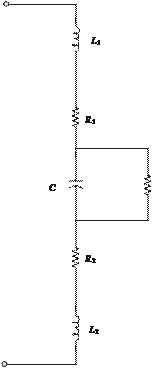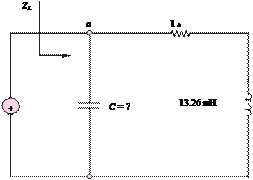### Create an Account

Already have account?

### Forgot Your Password ?

Home / Questions / Find the equivalent impedance ZL shown in Figure P466a as seen by the source if the freque...

# Find the equivalent impedance ZL shown in Figure P466a as seen by the source if the frequency is 377 rads b If we wanted the source to see the load as completely resistive what value of

Find the equivalent impedance Zshown in Figure P4.66(a), as seen by the source, if the frequency is 377 rad/s.

b.    If we wanted the source to see the load as completely resistive, what value of capacitance should we place between the terminals and as shown in Figure P4.66(b)? Hint: Find an expression for the equivalent impedance ZL, and then find so that the phase angle of the impedance is zero.

wire resistances, and L1 and L2 represent the lead wire inductances.

a.    If = 1 μF, R= 100 MQR1 = R2 = 1 μQ and L1 = L2 = 0.μH, find the equivalent impedance seen at the terminals and as a function of

frequency ω.

b.    Find the range of frequencies for which Zab is capacitive, i.e., Xab 10|Rab.

Hint: Assume that Ris is much greater than 1/wC so that you can replace RC  by an infinite resistance in part b.avS

b(a)

RC

Jun 19 2020 View more View Less

#### Answer (Solved)Subscribe To Get Solution